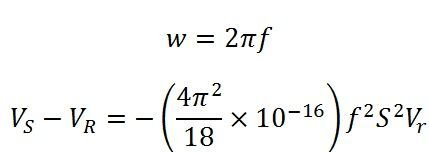# Ferranti Effect

Definition: The effect in which the voltage at the receiving end of the transmission line is more than the sending voltage is known as the Ferranti effect. Such type of effect mainly occurs because of light load or open circuit at the receiving end.

Ferranti effect is due to the charging current of the line. When an alternating voltage is applied, the current that flows into the capacitor is called charging current. A charging current is also known as capacitive current. The charging current increases in the line when the receiving end voltage of the line is larger than the sending end.

## Why Ferranti effect occurs?

Capacitance and inductance are the main parameters of the lines having a length 240km or above. On such transmission lines, the capacitance is not concentrated at some definite points. It is distributed uniformly along the whole length of the line.

When the voltage is applied at the sending end, the current drawn by the capacitance of the line is more than current associated with the load. Thus, at no load or light load, the voltage at the receiving end is quite large as compared to the constant voltage at the sending end.

### Detail explanation of the Ferranti effect by considering a nominal pi (π) model:

Let us consider the long transmission line in which OE represents the receiving end voltage; OH represent the current through the capacitor at the receiving end. The phasor FE represents the voltage drop across the resistance R. The voltage drop across the X (inductance). The phasor OG represents the sending end voltage under a no-load condition.It is seen from phasor diagram that OE > OG. In other words, the voltage at the receiving end is greater than the voltage at the sending end when the line is at no load.For a nominal pi (π) modelFor overhead lines, 1/√lc = velocity of propagation of electromagnetic waves on the transmission lines = 3×10^8m/s.Above equation shows that (VS-Vr ) is negative. That is Vr>VS. This equation also shows that Ferranti effect also depends on frequency and the electrical length of the lines.

In general, for any lineAt no load,For a long line, A is less than unity, and it decrease with the increase in the length of the line. Hence, the voltage at no load is greater than the voltage at no load (Vrnl > Vs). As the line length increases the rise in the voltage at the receiving end at no load becomes more predominant.

### How to reduce Ferranti effect:

Electrical devices are designed to work at some particular voltage. If the voltages are high at the user ends their equipment get damaged, and their windings burn because of high voltage. Ferranti effect on long transmission lines at low load or no load increases the receiving end voltage.This voltage can be controlled by placing the shunt reactors at the receiving end of the lines.

Shunt reactor is an inductive current element connected between line and neutral to compensate the capacitive current from transmission lines. When this effect occurs in long transmission lines, shunt reactors compensate the capacitive VAr of the lines and therefore the voltage is regulated within the prescribed limits.

### Note:

• Voltage rise is directly proportional to the square of the length of a line.
• Ferranti effect is more occurs in short transmission cables because their capacitance is high.

### 10 thoughts on “Ferranti Effect”

1. msana we ngombe

What is the purpose of installing reactors at the receiving end of a transmission line?

1. Reactors at the receiving end of the transmission line absorb the reactive power generated by the capacitance of the line.

2. Shanmukh puthineedi

By Installing reactors,we can control the voltage at the receiving end as less than the sending end voltage.

2. would shunt reactors be required on a 6 km 230kV XLPE line with 42 amp charging current & 6 MVAR ??
total capacitance 0.84 uF